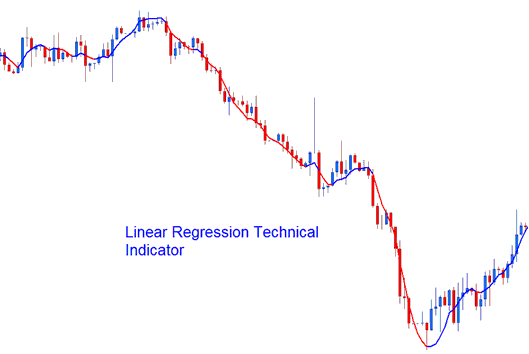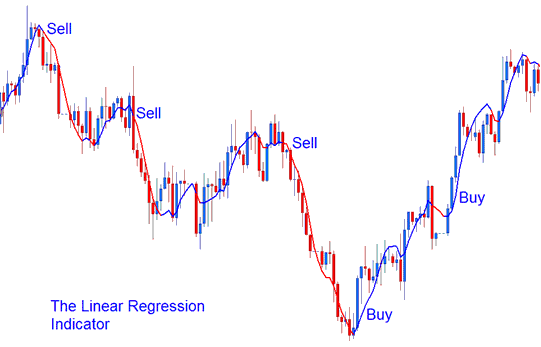# Linear Regression Indices Technical Analysis and Linear Regression Indices Trading Signals

Another name for a regression line is a line of the best fit/best fit line. This indicator plots the indices trend of the stock indexes price over a specified duration of time. The market indices trend is determined by calculating a Linear Regression Indices Trend Line using the "least squares fit" method. This method helps to minimize the distance between the stock indexes price data points and the line of best fit.Unlike the straight Regression Trend-Line indicator, the indicator plots the end values of multiple Linear Regression trend-lines. Any single point along the Linear Regression will be equal to the end value of a Regression Trend-line, but the resulting indices trend line looks like the Moving Average.

But unlike the Moving Average, this indicator does not show as much delay since it is fitting a line onto data points rather than averaging them.

The Linear Regression is a prediction of the tomorrow's stock indexes price drawn today, one day before. When the indices prices are steadily higher or lower than the forecast indices price, then a Indices trader can expect them to quickly return to more realistic fore-casted levels.

In other terms, this indicator shows where the chart indices prices "should" be trading based on a statistical basis and any disproportionate deviation from the regression line is likely to be short-lived.

## Indices Technical Analysis and Generating Indices Trading Signals

This indicator allows for stock indexes price selection, adjusting number of periods and smoothing of stock indexes price data before applying the calculation and the selection of smoothing type.

This indicator looks like a moving average but it has a bi-color representation.

• Bullish Signal (Blue color) - A rising line (greater than its previous value 1 previous bar before) is displayed in the up indices trend blue color, while

• Bearish Signal (Red color) - a declining line (lower than its previous value 1 previous bar before) is displayed in the down indices trend red color.Technical Analysis